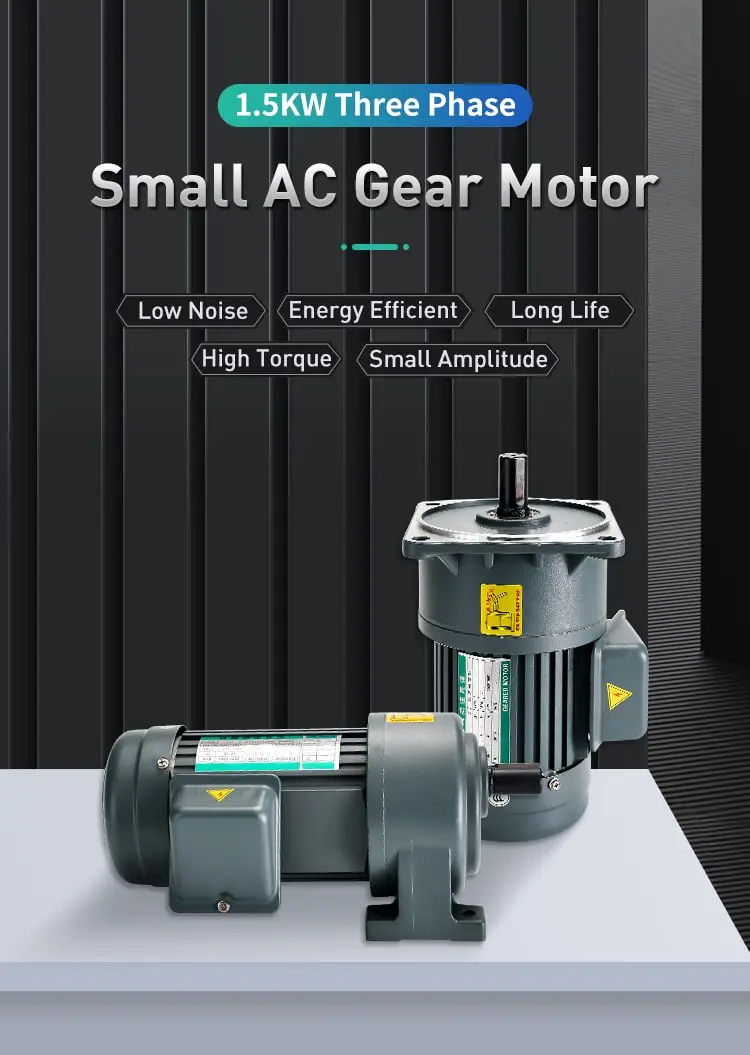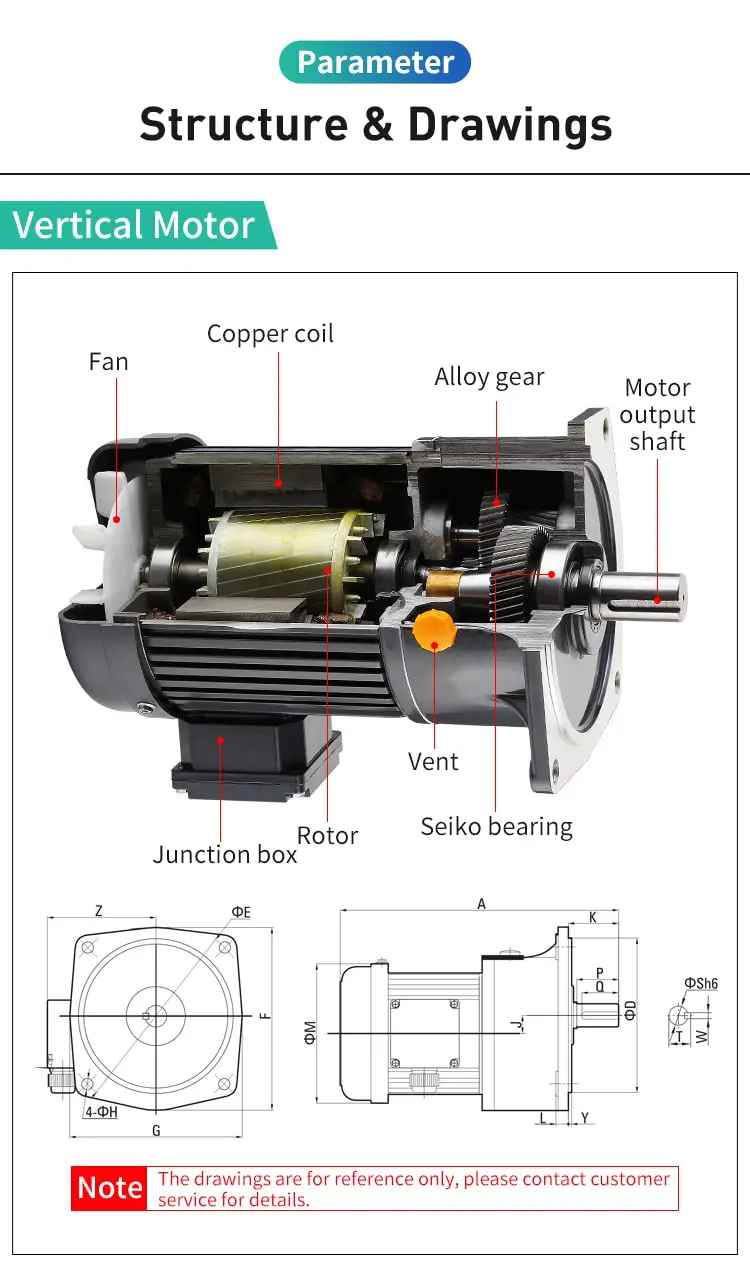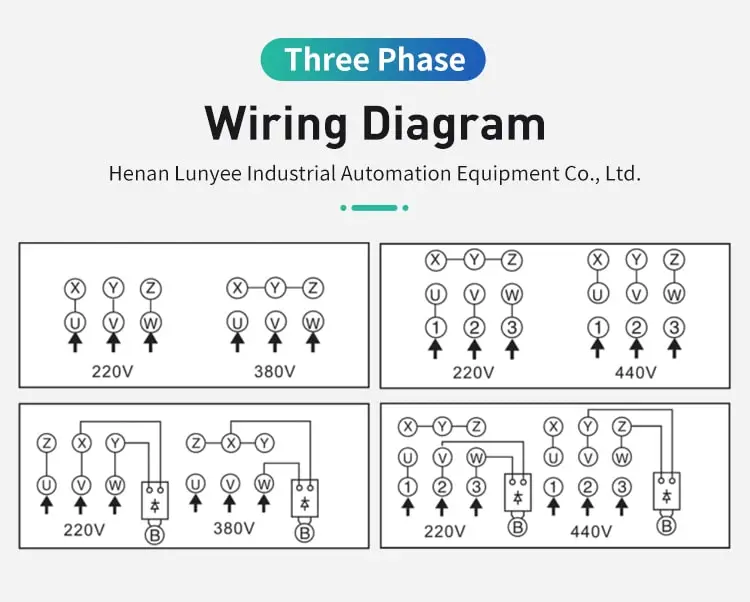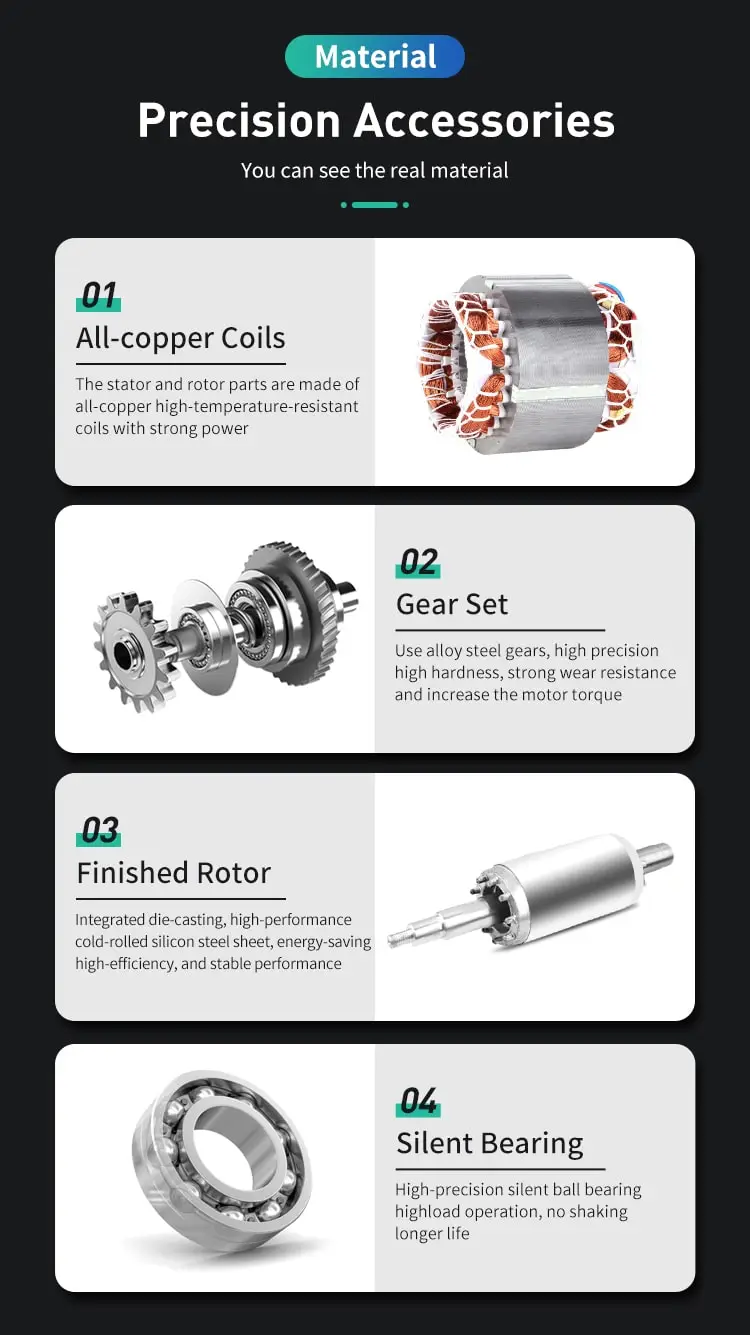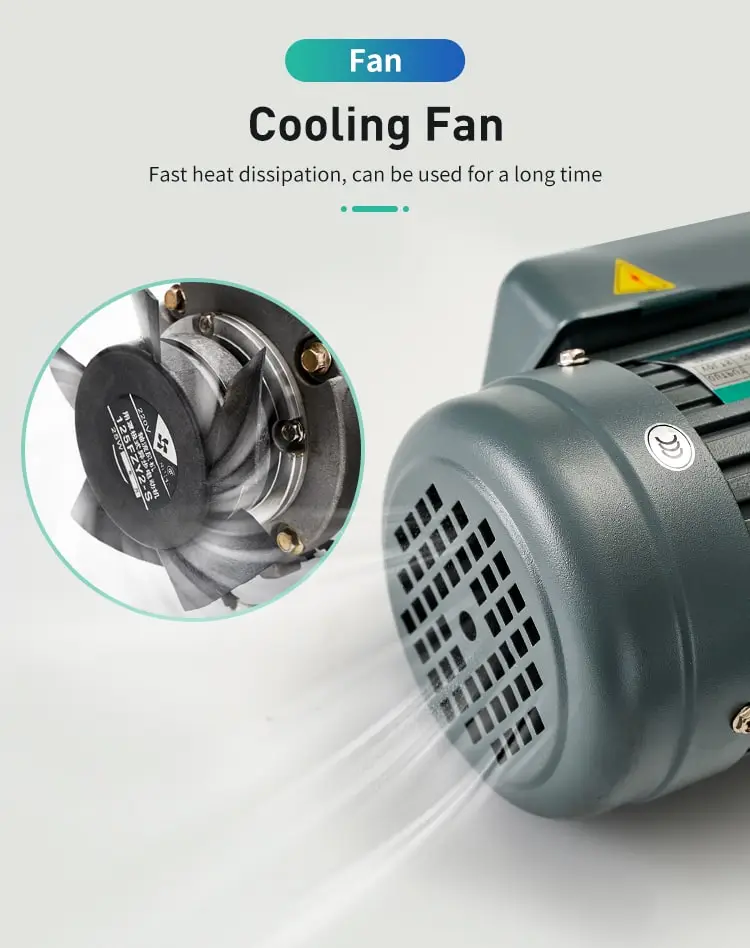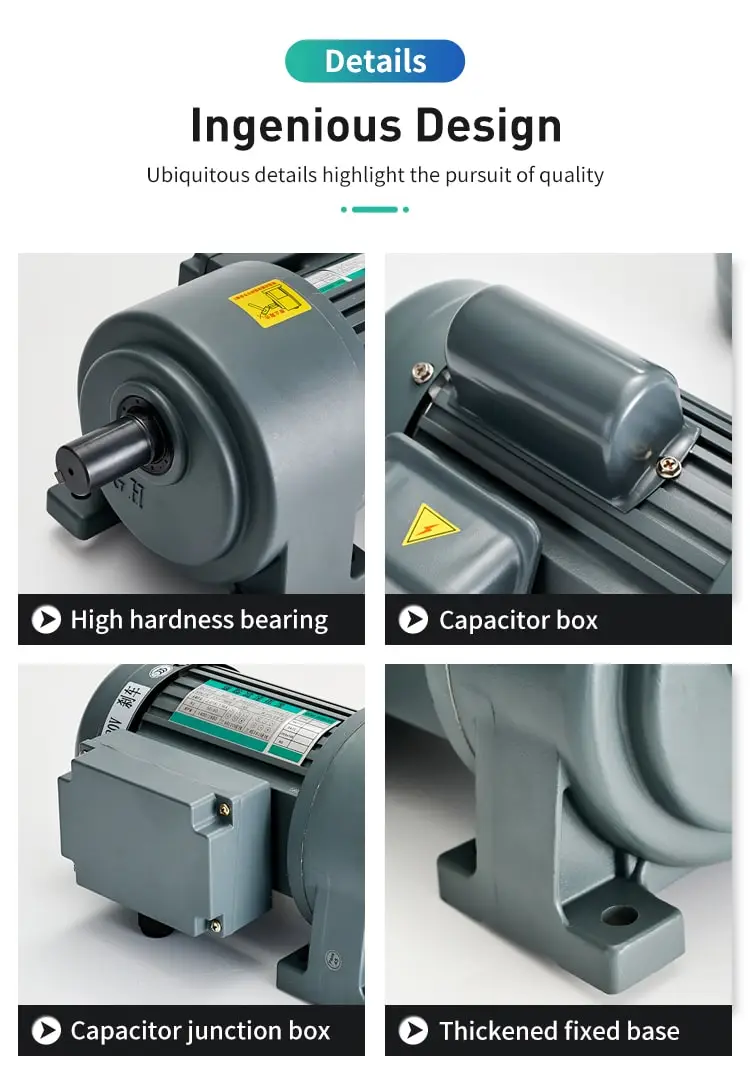By Jasmine 2023-03-02 11:43:38

#### How to calculate starting current of induction motor?

The starting current of an induction motor can be found in its technical specifications or measured using a current clamp meter. It is typically several times higher than its rated current. Soft-start or VFD technology can be used to limit the starting current and ensure smooth operation.

Calculating the starting current of an induction motor is an important step in determining its suitability for a particular application. The starting current is the current drawn by the motor when it is starting up from rest, and is typically much higher than the running current of the motor. This high starting current can cause issues with the electrical system and the drive components, such as belts and gears, and can even damage the motor itself if not properly managed.

To calculate the starting current of an induction motor, we need to know the following values:

1. Rated power of the motor: This is the power output of the motor when it is operating at its rated load and speed. It is typically expressed in horsepower (hp) or kilowatts (kW).

2. Rated voltage of the motor: This is the voltage at which the motor is designed to operate when it is at its rated load and speed. It is typically expressed in volts (V).

3. Efficiency of the motor: This is the ratio of the output power of the motor to the input power, expressed as a percentage. It indicates how much of the input power is converted into useful output power.

Once we have these values, we can use the following formula to calculate the starting current of the motor:

Starting current (Amps) = (Rated power (kW) x 1000) / (Rated voltage (V) x Efficiency (decimal))

For example, consider a three-phase induction motor with a rated power of 10 hp, a rated voltage of 480 V, and an efficiency of 80%. Using the formula above, we can calculate the starting current of the motor as follows:

Starting current (Amps) = (10 hp x 0.746 kW/hp x 1000) / (480 V x 0.8) = 16.01 Amps

It is important to note that the starting current of an induction motor is only valid when the motor is starting from rest. Once the motor reaches its rated speed, the current drawn by the motor will decrease to the running current. The running current of an induction motor is typically much lower than the starting current, and is determined by the load on the motor and the efficiency of the motor.

To determine the actual current drawn by the motor at a given load and speed, we need to know the speed-torque characteristic of the motor. This is a graph that shows the relationship between the speed of the motor and the torque produced by the motor. The speed-torque characteristic of an induction motor is typically a downward-sloping curve, with the torque decreasing as the speed increases.

To determine the actual current drawn by the motor at a given load and speed, we can simply find the point on the speed-torque characteristic curve that corresponds to the given load and speed, and use the efficiency of the motor to calculate the current drawn by the motor.

In conclusion, calculating the starting current of an induction motor is an important step in determining its suitability for a particular application. This value is determined using the rated power, voltage, and efficiency of the motor, and can be used to determine the actual current drawn by the motor at different loads and speeds using its speed-torque characteristic curve and the efficiency of the motor.